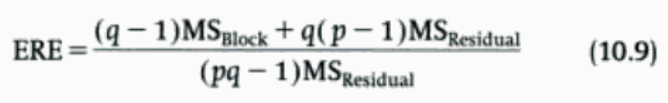# PMean: Estimating the efficiency of a completely randomized block design

I needed to look up a formula for the estimating the relative efficiency to a completely randomized block design to a design without blocking.

A quick search on Google revealed the formula:Here’s where I needed the formula. I was running a Monte Carlo simulation of three different statistical estimators. Each replication of the simulation required you to generate a binomial random variable with 100 trials and a probability of success of 0.5. You could just generate these values on the fly, but it makes sense to save the binomials that you used for one estimator and re-use the same ones for the other two estimators. This makes each replication in the Monte Carlo simulation a block, and you can estimate the precision that you have gained by the careful re-use of these binomials by fitting a completely randomized block design.

``` > tst.m1 <- lm(bb~trt+blk,data=mcd)
> anova(tst.m1)
Analysis of Variance Table
Response: bb
Df  Sum Sq Mean Sq   F value    Pr(>F)
trt          2 11.5617  5.7809 35659.326 < 2.2e-16 ***
blk        999  2.2959  0.0023    14.176 < 2.2e-16 ***
Residuals 1998  0.3239  0.0002
---
Signif. codes:  0 ‘***’ 0.001 ‘**’ 0.01 ‘*’ 0.05 ‘.’ 0.1 ‘ ’ 1
> (999*0.0023+2000*0.0002)/(2999*0.0002)
 4.497666```

The re-use of the binomials has resulted in a 4.5 fold improvement in precision.

This formula was published in Experimental Design and Data Analysis for Biologists by Gerald Peter Quinn, Michael J. Keough, (ISBN: 9780521009768) and digitized by Google. There is a huge controversy over the  Google project to digitize a whole bunch of books and make them accessible through their search engine. But I have to admit that I’m glad to have access to all those books for finding a formula like this.